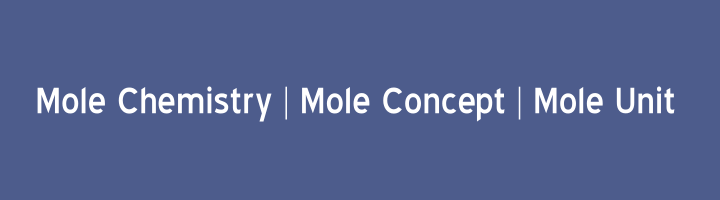Below are some important points to get clear mole concept, definition of mole chemistry and mole units.

Mole can be define as,

The atomic mass, molecular mass, ionic mass or formula mass of a substance expressed in grams is called mole of that substance.

OR

The amount of a substance, which contains 6.02 x 1023 atoms, molecules, ions or formula units is called mole of that substance.

OR

The amount, which contains Avogadro’s number of units i.e., atoms, molecules, ions, formula units or what is under consideration is called mole.

## Explanation of Mole Chemistry:

We can use mole concept with other terminologies also for different types of substances like elements, molecular compounds, ionic compounds & ions.

Following are different terms and example which will explain the mole concept or mole chemistry with mole units in easiest & explanatory way.

### Gram Atomic Mass Definition:

Atomic mass of an element expressed in gram is called gram atomic mass or gram atom or mole of the element.

Examples:

(i) Atomic mass of hydrogen = 1.008

1 gram atom of hydrogen = Molar mass of hydrogen =1.008 g = 1mole

(ii) Atomic mass of carbon = 12.000

1 gram atom of carbon = Molar mass of carbon = 12 g = 1mole

(iii) Atomic mass of magnesium= 24

1 gram atom of magnesium = Molar mass of magnesium = 24 g= 1mole

It is clear from the above that one gram atom of different elements has different masses.

### Gram Molecular Mass Definition:

The molecular mass of a substance expressed in grams is called gram molecular mass or gram molecule or mole of the substance.

Examples:

(i) Molecular mass of H2 = 2.016

1 gram molecule of H2 = Molar mass of H2 = 2.016 g = 1mole

(ii) Molar mass of H2O = 181

1 gram molecule of H2O = Molar mass of H2O = 18 g = 1mole

(iii) Molecular mass of CO2 = 44

1 gram molecule of CO2 = Molar mass of CO2 = 44 g = 1mole

It is clear from the above that one gram molecule of different molecules justify that different substances has different masses.

### Gram Formula Definition:

The formula mass of an ionic compound expressed in grams is called gram formula or mole of the substance.

Examples:

(i) Formula mass of NaCl = 58.5

1 gram formula of NaCl = Molar mass of NaCl = 58.5 g = 1mole

(ii) Formula mass of NaOH = 40

1 gram formula of NaOH = Molar mass of NaOH = 40 g = 1mole

### Gram lon Definition:

The ionic mass of an ionic species expressed in grams is called gram ion or mole of the ion.

Examples:

(i) Ionic mass of Na+1 = 23

1 gram ion of Na+1 = Molar mass of Na+1 = 23 g = 1mole

(ii) Ionic mass of OH-1 = 17

1 gram ion of OH-1= Molar mass of OH-1 = 17 g = 1mole